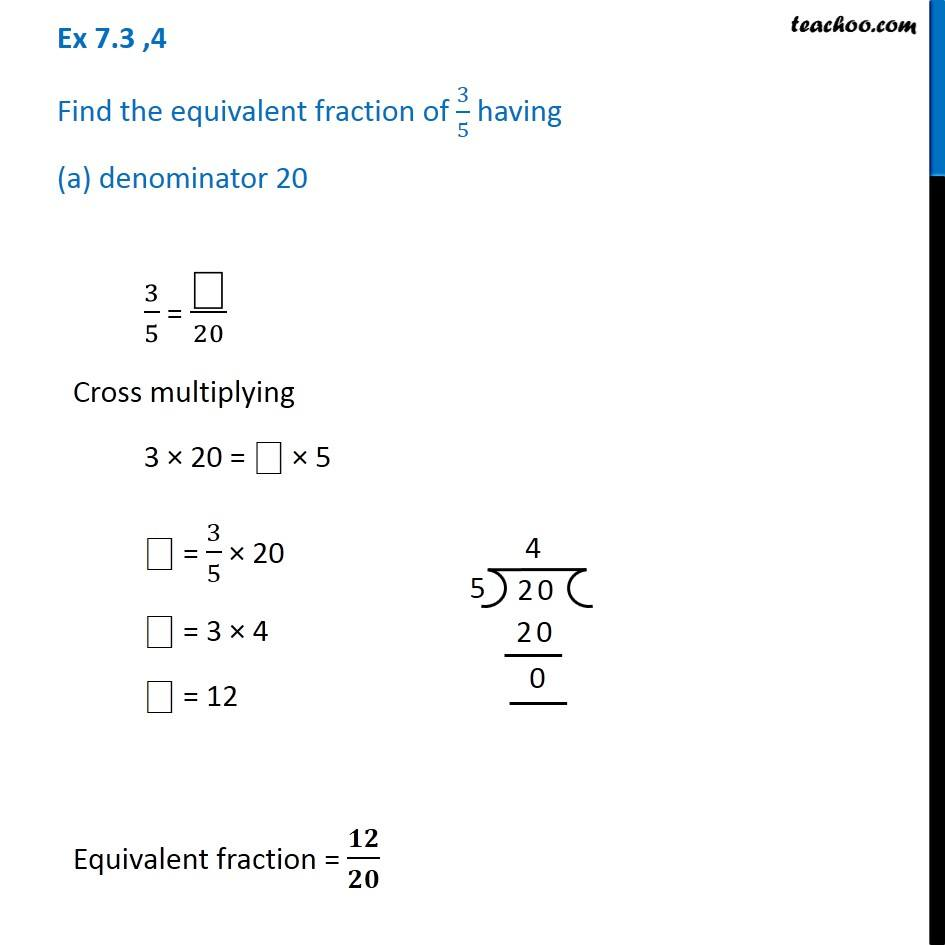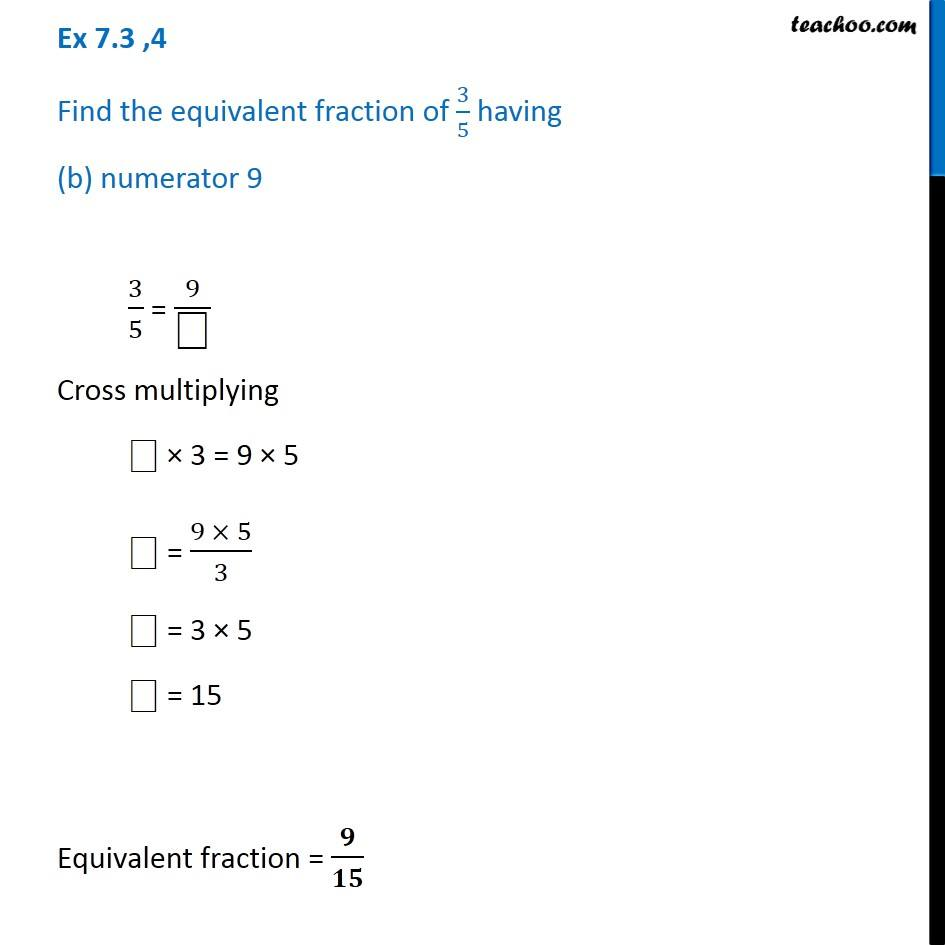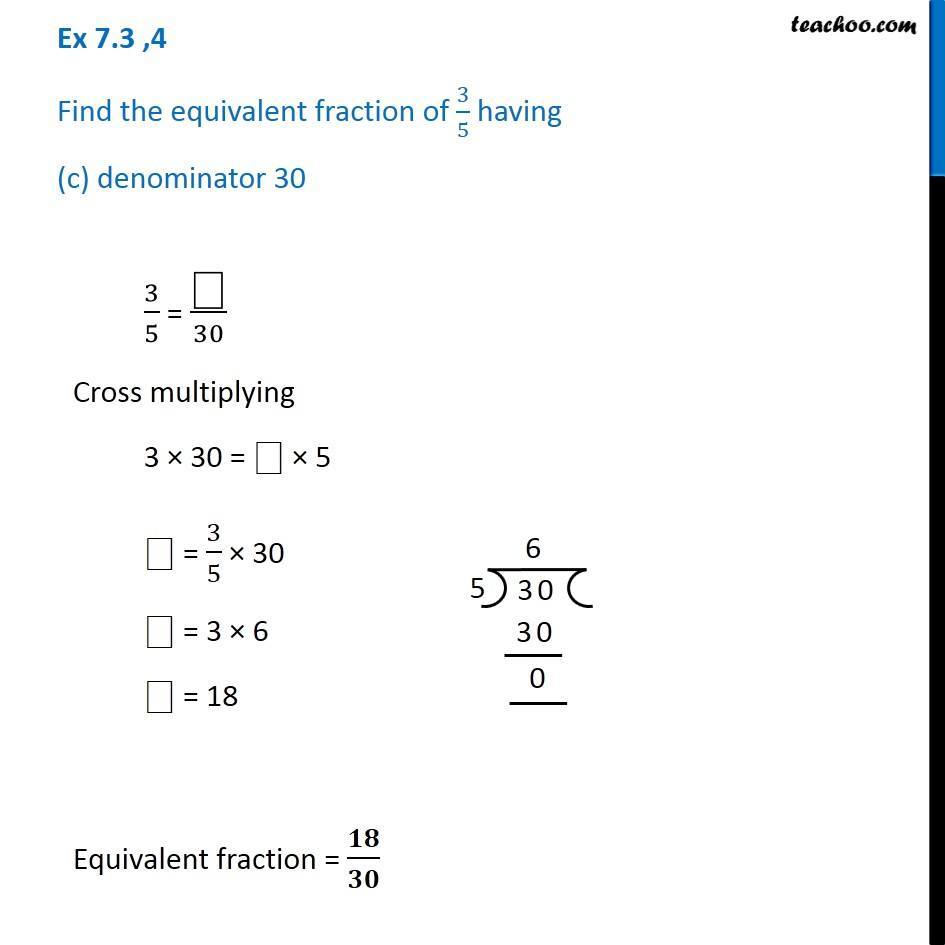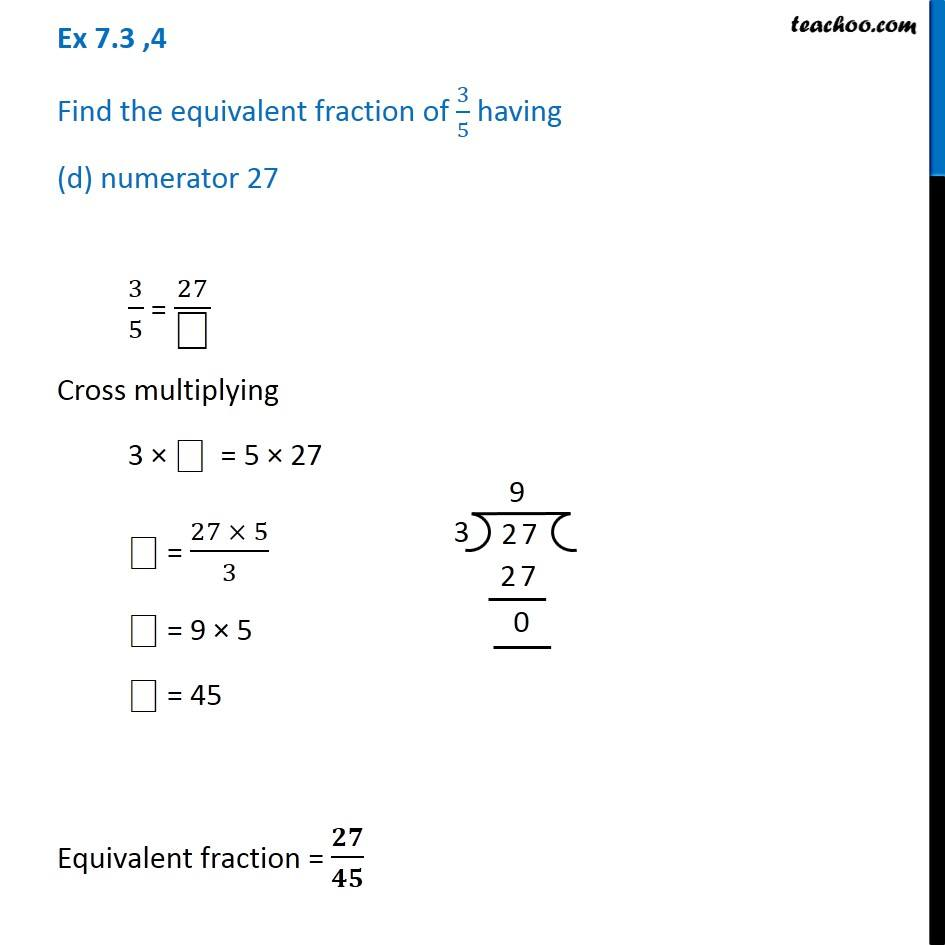Equivalent Fractions

Chapter 7 Class 6 Fractions
Concept wiseIntroducing your new favourite teacher - Teachoo Black, at only ₹83 per month

### Transcript

Ex 7.3 ,4 Find the equivalent fraction of 3/5 having (a) denominator 20 3/5 = "⎕" /20 Cross multiplying 3 × 20 = "⎕" × 5 "⎕" = 3/5 × 20 "⎕" = 3 × 4 "⎕" = 12 Equivalent fraction = 𝟏𝟐/𝟐𝟎 Ex 7.3 ,4 Find the equivalent fraction of 3/5 having (b) numerator 9 3/5 = 9/"⎕" Cross multiplying ⎕ × 3 = 9 × 5 "⎕" = (9 × 5)/3 "⎕" = 3 × 5 "⎕" = 15 Equivalent fraction = 𝟗/𝟏𝟓 Ex 7.3 ,4 Find the equivalent fraction of 3/5 having (c) denominator 30 3/5 = "⎕" /30 Cross multiplying 3 × 30 = "⎕" × 5 "⎕" = 3/5 × 30 "⎕" = 3 × 6 "⎕" = 18 Equivalent fraction = 𝟏𝟖/𝟑𝟎 Ex 7.3 ,4 Find the equivalent fraction of 3/5 having (d) numerator 27 3/5 = 27/"⎕" Cross multiplying 3 × ⎕ = 5 × 27 "⎕" = (27 × 5)/3 "⎕" = 9 × 5 "⎕" = 45 Equivalent fraction = 𝟐𝟕/𝟒𝟓